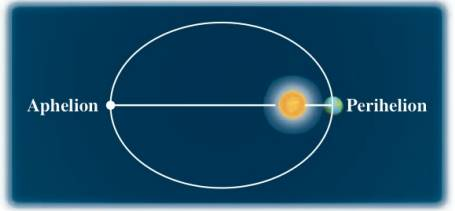Chapter 12.2, Problem 65E### Algebra and Trigonometry (MindTap ...

4th Edition
James Stewart + 2 others
ISBN: 9781305071742

#### Solutions

Chapter
Section### Algebra and Trigonometry (MindTap ...

4th Edition
James Stewart + 2 others
ISBN: 9781305071742
Textbook Problem

# APPLICATIONSPerihelion and Aphelion The planets move around the sun in elliptical orbits with the sun at one focus. The point in the orbits at which the planet is closest to the sun is called perihelion, and the point at which it is farthest is called aphelion. These points are the vertices of the orbit. The earth’s distance from the sun is 147 , 000 , 000  km at perihelion and 153 , 000 , 000  km at aphelion. Find an equation for the earth’s orbit. (Place the origin at the center of the orbits with the sun on the x -axis.)To determine

To Find:

The equation for the earth’s orbit.

Explanation

Given:

Figure (1)

The distance between earth and sun at perihelion is 147×106 km and distance between earth and sun at aphelion is 153×106 km.

Approach:

Let the point A(a,0) be perihelion, B(a,0) be aphelion and C(c,0) be the position of the sun.

Equation of ellipse with foci at (c,0) and (c,0), major axis 2a and minor axis 2b is:

x2a2+y2b2=1 ……(1)

Calculation:

The distance between a and c is given by,

ac=147×106 ……(2)

The distance between a and c is given by,

a(c)=153×106a+c=153×106 ……(3)

Solve equation (2) and (3),

### Still sussing out bartleby?

Check out a sample textbook solution.

See a sample solution

#### The Solution to Your Study Problems

Bartleby provides explanations to thousands of textbook problems written by our experts, many with advanced degrees!

Get Started